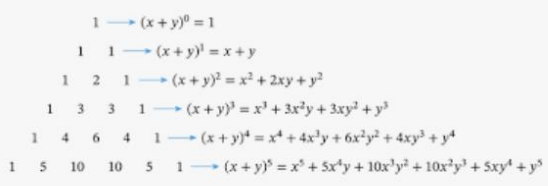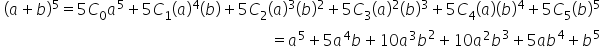Maths-
General
Easy

Question

# How are Pascal’s triangle and binomial expansion such as (a + b)5 related?Hint:

## The correct answer is: (x + y)n

### Step 1 of 1:Pascal's Triangle is a method to know the binomial coefficients of terms of binomial expression (x + y)n , where n can be any positive integer and x, y are real numbers. Pascal Triangle is represented in a triangular form, it is kind of a number pattern in the form of a triangular arrangement.The binomial expansion of (a + b)5 is:Here, the coefficients of the expansions are the elements of the sixth row of the Pascal’s triangle.

You can find the expansion of (x + y)n  using both Pascal’s triangle and binomial expansion.#### With Turito Foundation.#### Get an Expert Advice From Turito.